Get inspired by the success stories of our students in IIT JAM MS, ISI  MStat, CMI MSc DS.  Learn More

# ISI MStat 2018 PSA Problem 7 | Continuous FunctionThis is a problem from ISI MStat 2018 PSA Problem 7 based on Continuity.

## Continuous Function - ISI MStat Year 2018 PSA Question 7

Let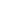be a function defined from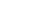to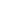such that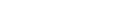and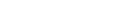for all x
Thenis

• (A) continuous and bounded.
• (B) continuous but not necessarily bounded.
• (C) bounded but not necessarily continuous.
• (D) neither necessarily continuous nor necessarily bounded.

### Key Concepts

Epsilon-Delta definition of limit

Continuity

Bounded function

ISI MStat 2018 PSA Problem 7

Introduction to Real Analysis by Bertle Sherbert

## Try with Hints

Try to use the epsilon-delta definition of limit and the property thatfor all x.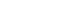such that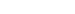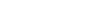. Nowfor all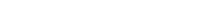for every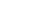.

Let's try to use this .

Given any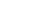we can select a suitablesuch that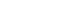. Then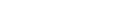. But. Hence ,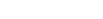. Hence , for all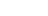we have. Since ,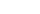is arbitrary , we must have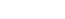for allSois continuous and bounded

## Subscribe to Cheenta at Youtube

This is a problem from ISI MStat 2018 PSA Problem 7 based on Continuity.

## Continuous Function - ISI MStat Year 2018 PSA Question 7

Letbe a function defined fromtosuch thatandfor all x
Thenis

• (A) continuous and bounded.
• (B) continuous but not necessarily bounded.
• (C) bounded but not necessarily continuous.
• (D) neither necessarily continuous nor necessarily bounded.

### Key Concepts

Epsilon-Delta definition of limit

Continuity

Bounded function

ISI MStat 2018 PSA Problem 7

Introduction to Real Analysis by Bertle Sherbert

## Try with Hints

Try to use the epsilon-delta definition of limit and the property thatfor all x.such that. Nowfor allfor every.

Let's try to use this .

Given anywe can select a suitablesuch that. Then. But. Hence ,. Hence , for allwe have. Since ,is arbitrary , we must havefor allSois continuous and bounded

## Subscribe to Cheenta at Youtube

This site uses Akismet to reduce spam. Learn how your comment data is processed.

### Knowledge Partner